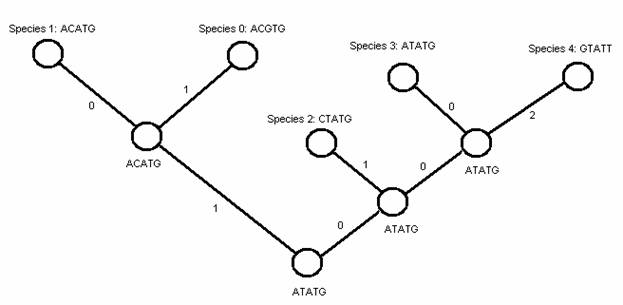Имя: Пароль: Зарегистрироваться Восстановить пароль
10/12/2023 03:24:24

#Рабочее место участника

##Задачи

982. Ancestor

Ограничения: время – 1s/2s, память – 16MiB Ввод: input.txt или стандартный ввод Вывод: output.txt или стандартный выводПослать решение Blockly Посылки Темы Где Обсудить (0)

Reconstructing the evolutionary relationships among species is one of major subjects in biology. Typically, each species is presented by a sequence over four nucleotide types: A, C, G, and T. A nucleotide mutation is said to be happened at position i between two sequences X\ =\ (x_1,\ …,\ x_l) and Y\ =\ (y_1,\ …,\ y_l) if x_i\ ≠\ y_i. The distance between two sequences X and Y is calculated as the total number of nucleotide mutations between them.
There are n contemporary species, which are labeled from 0 to n-1. The evolutionary relationships among species are depicted by a binary rooted tree where n leaves represent n contemporary species, internal nodes represent ancestor species, and branch lengths represent distances between species (see figure below). This tree can be represented in text form using brackets as ((0,1),((3,4),2)).Since nucleotide sequences are not available for ancestor species, our task is to determine one nucleotide sequence for each ancestor species such that the tree length (total branch lengths) is minimized.
Input
The first line containing two integers n and l (n\ ≤\ 1000, l\ ≤\ 100) indicating the number of contemporary species and the length of nucleotide sequences, respectively. The i-th line of the following n lines contains the nucleotide sequence of the contemporary species labeled i-1. The last line contains the text representation of the tree topology.
Output
Write an integer number indicating the minimum length of the tree.

Sample Input 1

2 3
AGG
CGT
(0,1)


Sample Output 1

2


Sample Input 2

5 5
ACGTG
ACATG
CTATG
ATATG
GTATT
((0,1),(2,(3,4)))


Sample Output 2

5

Источник: РГУ им. И.Канта, осенний командный турнир, 2007